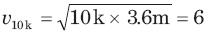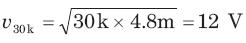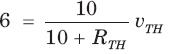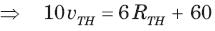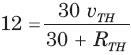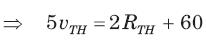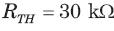Courses

# Networks Theorem - MCQ Test

## 20 Questions MCQ Test GATE ECE (Electronics) 2022 Mock Test Series | Networks Theorem - MCQ Test

Description
This mock test of Networks Theorem - MCQ Test for Railways helps you for every Railways entrance exam. This contains 20 Multiple Choice Questions for Railways Networks Theorem - MCQ Test (mcq) to study with solutions a complete question bank. The solved questions answers in this Networks Theorem - MCQ Test quiz give you a good mix of easy questions and tough questions. Railways students definitely take this Networks Theorem - MCQ Test exercise for a better result in the exam. You can find other Networks Theorem - MCQ Test extra questions, long questions & short questions for Railways on EduRev as well by searching above.
QUESTION: 1

### vTH , RTH = ?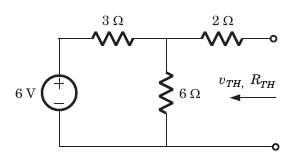Solution: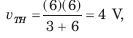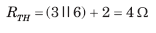QUESTION: 2

### IN  , RN = ?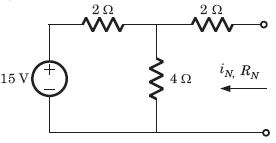Solution: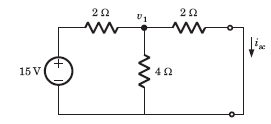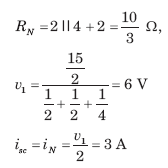QUESTION: 3

### Which theorem assists in replacement of an impedance branch over the network by the other network comprising different circuit components, without affecting the V-I relations throughout the entire network?

Solution:
QUESTION: 4

A simple equivalent circuit of the 2 terminal network shown in fig.  is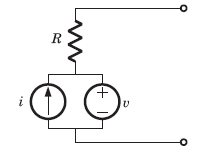Solution:

After killing all source equivalent resistance is R
Open circuit voltage = v1

QUESTION: 5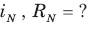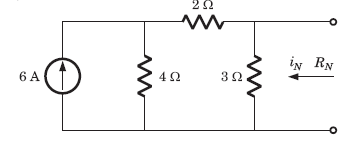Solution:

The short circuit current across the terminal is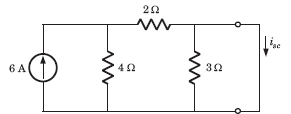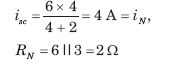QUESTION: 6

VTH, RTH  = ?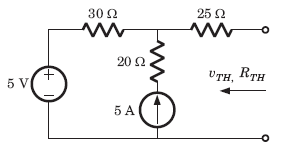Solution:

For the calculation of RTH if we kill the sources
then 20Ω resistance is inactive because 5 A source will
be open circuit
RTH = 30+25 = 55 Ω,
vTH = 5+5X30 = 155V

QUESTION: 7

RTH = ?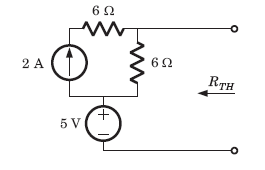Solution:

After killing the source, RTH = 6 Ω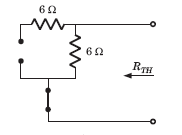QUESTION: 8

The Thevenin impedance across the terminals ab of the network shown in fig. is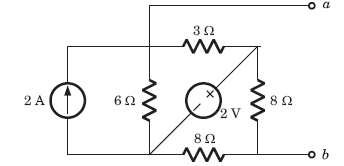Solution:

After killing all source,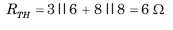QUESTION: 9

For In the the circuit shown in fig. a network and its Thevenin and Norton equivalent are given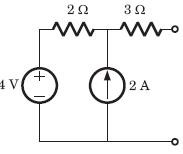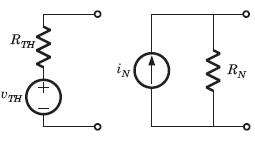The value of the parameter are

VTH  RTH  I RRN

Solution:

v∝ = 2 x 2 + 4 = 8 V = vTH
RTH = 2 + 3 = 5Ω = RN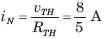QUESTION: 10

v1 = ?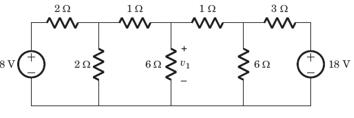Solution:

If we solve this circuit direct, we have to deal with three variable. But by simple manipulation variable can be reduced to one. By changing the LHS and RHS in Thevenin equivalent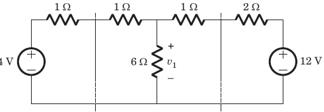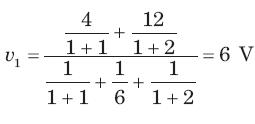QUESTION: 11

i1 =  ?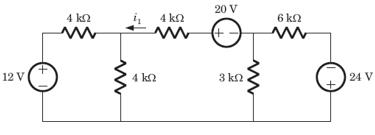Solution:

If we solve this circuit direct, we have to deal with three variable. But by simple manipulation variable can be reduced to one. By changing the LHS and RHS in Thevenin equivalent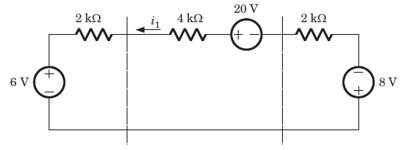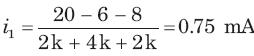QUESTION: 12

A circuit is given in fig.  Find the Thevenin equivalent as given in question..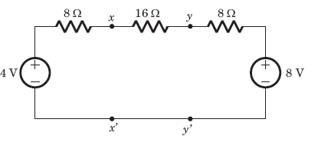As viewed from terminal x and x' is

Solution:

We Thevenized the left side of xx'and source transformed right side of yy'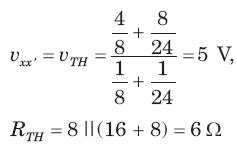QUESTION: 13

A circuit is given in fig. Find theThevenin equivalent as given in question.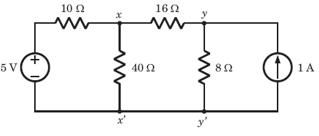As viewed from terminal y and y' is

Solution:

Thevenin equivalent seen from terminal yy' is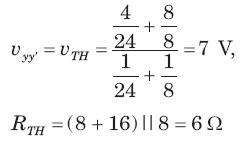QUESTION: 14

A practical DC current source provide 20 kW to a 50Ω load and 20 kW to a 200 Ω load. The maximum power, that can drawn from it, is

Solution: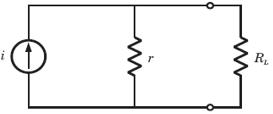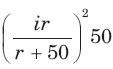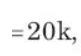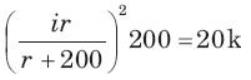(r + 200)2 = 4(r + 50)2
⇒ r = 100 Ω
i = 30A,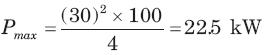QUESTION: 15

In the circuit of fig. P.1.4.15–16 when R = 0 Ω , the current iR equals 10 A.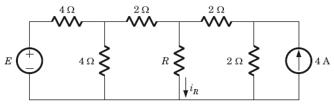The value of R, for which it absorbs maximum power, is

Solution:

Thevenized the circuit across R,RTH = 2 Ω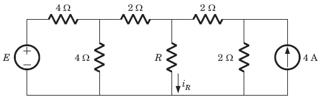QUESTION: 16

In the circuit of fig when R = 0 Ω , the current iR equals 10 A.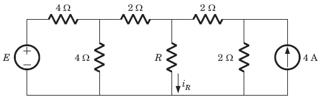The maximum power will be

Solution: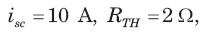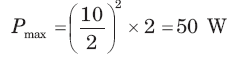QUESTION: 17

A battery has a short-circuit current of 30 A and an open circuit voltage of 24 V. If the battery is connected to an electric bulb of resistance 2Ω, the power dissipated by the bulb is

Solution:

rbattery VocIsc = 24/30 = 0.8 ohms

P = V2oc / ( r + 2)2   * 2

= 24/ ( .8 + 2)2  * 2

= 146.9 W

QUESTION: 18

The following results were obtained from measurements taken between the two terminal of a resistive network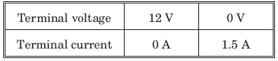The Thevenin resistance of the network is

Solution: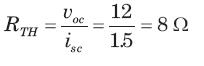QUESTION: 19

A DC voltmeter with a sensitivity of 20 kΩ/V is used to find the Thevenin equivalent of a linear network. Reading on two scales are as follows

(a) 0 - 10 V scale  : 4 V
(b) 0 - 50 V scale : 5 V

The Thevenin voltage and the Thevenin resistance of the network is

Solution: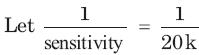= 50 μ A

For 0 - 10 V scale Rm = 10 x 20k = 200 kΩ
For 0 - 50 V scale Rm = 50 x 20k = 1 MΩ
For 4 V reading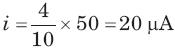vTH = 20μRTH + 20µ x 200k = 4 + 20μRTH     ...(i)
For 5V reading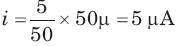UTH = 5μ x RTH + 5μ x 1M = 5 + 5μRTH        ...(ii)
Solving (i) and (ii)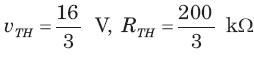QUESTION: 20

Consider the network shown in fig.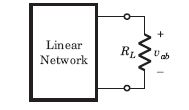The power absorbed by load resistance RL is shown in table :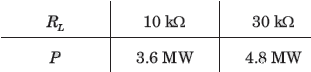Find the Thevenin equivalent.

Solution: